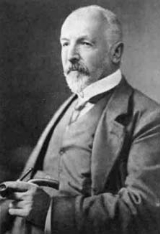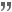xGeorg CantorOverview

Georg Ferdinand Ludwig Philipp Cantor (icon ; ɡeˈɔʁk ˈfɛʁdinant ˈluːtvɪç ˈfiːlɪp ˈkantɔʁ; – January 6, 1918) was a German
Germans
The Germans are a Germanic ethnic group native to Central Europe. The English term Germans has referred to the German-speaking population of the Holy Roman Empire since the Late Middle Ages....

mathematician
Mathematician
A mathematician is a person whose primary area of study is the field of mathematics. Mathematicians are concerned with quantity, structure, space, and change....

, best known as the inventor of set theory
Set theory
Set theory is the branch of mathematics that studies sets, which are collections of objects. Although any type of object can be collected into a set, set theory is applied most often to objects that are relevant to mathematics...

, which has become a fundamental theory
Foundations of mathematics
Foundations of mathematics is a term sometimes used for certain fields of mathematics, such as mathematical logic, axiomatic set theory, proof theory, model theory, type theory and recursion theory...

in mathematics. Cantor established the importance of one-to-one correspondence between the members of two sets, defined infinite and well-ordered sets
Well-order
In mathematics, a well-order relation on a set S is a strict total order on S with the property that every non-empty subset of S has a least element in this ordering. Equivalently, a well-ordering is a well-founded strict total order...

, and proved that the real number
Real number
In mathematics, a real number is a value that represents a quantity along a continuum, such as -5 , 4/3 , 8.6 , √2 and π...

s are "more numerous" than the natural number
Natural number
In mathematics, the natural numbers are the ordinary whole numbers used for counting and ordering . These purposes are related to the linguistic notions of cardinal and ordinal numbers, respectively...

s.Quotations

In re mathematica ars proponendi pluris facienda est quam solvendi.In mathematics the art of asking questions is more valuable than solving problems.

I realize that in this undertaking I place myself in a certain opposition to views widely held concerning the mathematical infinite and to opinions frequently defended on the nature of numbers.Grundlagen einer allgemeinen Mannigfaltigkeitslehre [Foundations of a General Theory of Aggregates] (1883)

Had Mittag-Leffler had his way, I should have to wait until the year 1984, which to me seemed too great a demand!Letter (1885), written after Gösta Mittag-Leffler|Gösta Mittag-Leffler persuaded him to withdraw a submission to Mittag-Leffler's journal Acta Mathematica, telling him it was "about one hundred years too soon."

There is no doubt that we cannot do without variable quantities in the sense of the potential infinite. But from this very fact the necessity of the actual infinite can be demonstrated."Über die verschiedenen Ansichten in Bezug auf die actualunendlichen Zahlen" ["Over the different views with regard to the actual infinite numbers"] - Bihand Till Koniglen Svenska Vetenskaps Akademiens Handigar (1886)

The potential infinite means nothing other than an undetermined, variable quantity, always remaining finite, which has to assume values that either become smaller than any finite limit no matter how small, or greater than any finite limit no matter how great."Mitteilungen" (1887-8)

Er ist aber in Kopenhagen geboren, von israelitischen Eltern, die der dortigen portugisischen Judengemeinde.However, he was born in Copenhagen, of Jewish parents, of the Portuguese Jewish community there.